# Back Adjusted Continuous Contract Data from Quandl

I finally got around to programming an easy Python down loader for CME data from Quandl.  I promised this in my last book, but haven’t got that many requests.   On the Ultimate Algorithmic Trading System…. page I have provided some data going back to early 2008 on several markers.  This is a work in progress.  I also wrote a back adjuster that rolls when the Open Interest + Volume is greater in the next contract and adjusts the data retroactively (Panama process) by the discount on the roll date.  As I state on the web page this data is not all that great even though it looks like it comes directly from CME.   I do fill in gaps and try to fix glaring errors so take it for what it is.  Its good data for preliminary testing, but to finalize an algorithm or to trade by I would definitely go the “paid” route.  Although the later data looks pretty good.

Before downloading the data I would sign up for Quandl and get an API key.  You will be amazed at the amount of data they make available – free and paid subscriptions.

# Anatomy Of Mean Reversion in EasyLanguage

Look at this equity curve: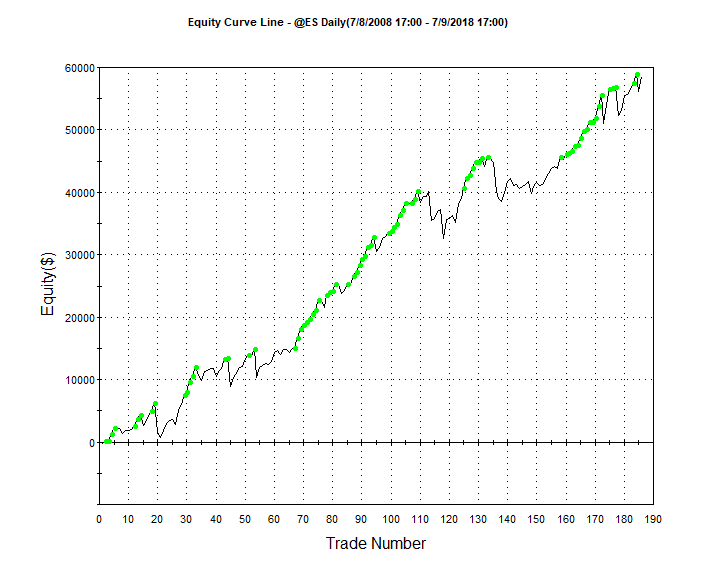As long as you are in a bull market buying dips can be very consistent and profitable.  But you want to use some type of entry signal and trade management other than just buying a dip and selling a rally.  Here is the anatomy of a mean reversion trading algorithm that might introduce some code that you aren’t familiar.  Scroll through the code and I will  summarize below.

``````inputs: mavlen(200),rsiLen(2),rsiBuyVal(20),rsiSellVal(80),holdPeriod(5),stopLoss\$(4500);

Condition1 = c > average(c,mavLen);

Condition3 = rsi(c,rsiLen) > rsiSellVal;

Value1 = 0;
Value2 = 0;

For iCnt = 0 to consecUpClose - 1
Begin
value1 = value1 + iff(c[iCnt] > c[iCnt+1],1,0);
end;

For iCnt = 0 to consecDnClose - 1
Begin
Value2 = value2 + iff(c[iCnt] < c[iCnt+1],1,0);
end;

dontCatchFallingKnife = absValue(C - c) < avgTrueRange(10)*2.0;

meanRevBuy = condition1 and condition2 and dontCatchFallingKnife;
meanRevSell =  not(condition1) and condition3 and dontCatchFallingKnife;

If marketPosition = 1 and condition1 and value1 >= consecUpClose then sell("ConsecUpCls") this bar on close;

If meanRevSell then sellShort this bar on close;
If marketPosition = -1 and not(condition1) and value2 >= consecDnClose then buyToCover this bar close;

setStopLoss(stopLoss\$);

Begin
if marketPosition = 1 and not(meanRevBuy) then sell this bar on close;
if marketPosition =-1 and not(meanRevSell) then buytocover this bar on close;
end;``````
Mean Reversion System

I am using a very short term RSI indicator, a la Connors, to initiate long trades.  Basically when the 2 period RSI dips below 30 and the close is above the 200-day moving average I will buy only if I am not buying “a falling knife.”  In February several Mean Reversion algos kept buying as the market fell and eventually got stopped out with large losses.  Had they held on they probably would have been OK.  Here I don’t buy if the absolute price difference between today’s close and yesterday’s is greater than 2 X the ten day average true range.  Stay away from too much “VOL.”

Once a trade is put on I use the following logic to keep track of consecutive closing relationships:

``````For iCnt = 0 to consecUpClose - 1
Begin
value1 = value1 + iff(c[iCnt] > c[iCnt+1],1,0);
end;``````
Using the IFF function in EasyLanguage

Here I am using the IFF function to compare today’s close with the prior day’s.  iCnt is a loop counter that goes from 0 to 1. IFF checks the comparison and if it’s true it returns the first value after the comparison and if false it returns the last value.  Here if I have two consecutive up closes value1 accumulates to 2.  If I am long and I have two up closes I get out.  With this template you can easily change this by modifying the input:  consecUpClose.  Trade management also includes a protective stop and a time based exit.  If six days transpire without two up closes then the system gets out – if the market can’t muster two positive closes, then its probably not going to go anywhere.  The thing with mean reversion, more so with other types of systems, is the use or non use of a protective stop.  Wide stops are really best, because you are betting on the market to revert.  Look at the discrepancy of results using different stop levels on this system: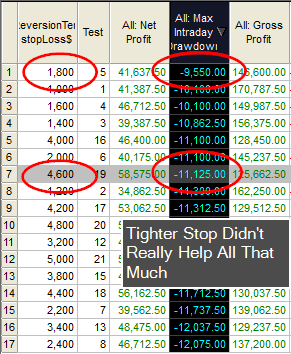Here an \$1,800 stop only cut the max draw down by \$1,575.  But it came at a cost of \$17K in profit.  Stops, in the case of Mean Reversion, are really used for the comfort of the trader.

This code has the major components necessary to create a complete trading system.  Play around with the code and see if you can come up with a better entry mechanism.

# Update To Original Pattern Smasher

## What will you learn : string manipulation, for-loops, optimization

Before proceeding I would suggest reading my original post on this subject.    If you believe the relationship of the last few bars of data can help determine future market direction, then this post will be in you wheel house.  Another added benefit is that you will also learn some cool EasyLanguage.

### Original post was limited to four day patterns!

This version is limitless (well not really, but pretty close).  Let’s stick with the original string pattern nomenclature (+ + – – : two up closes followed by two down closes.)  Let’s also stick with our binary pattern representation:

 Pattern # 2^3 2^2 2^1 1 3 0 0 1 1 4 0 1 0 0 5 0 1 0 1 6 0 1 1 1

Remember a 0 represents a down close and a 1 represents an up close.  We will deviate from the original post by doing away with the array and stick with only strings (which are really just arrays of characters.)  This way we won’t have to worry about array manipulation.

#### How to create a dynamic length string pattern

This was the difficult part of the programming.  I wanted to be able to optimize 3, 4 and 5 day patterns and I wanted to control this with using just inputs.  I discovered that pattern three is different in a three day pattern than it is in a four day pattern: in a three day pattern it is 011 or – + + and in a four day pattern it is 0011 or – – + +.  Since I am counting 0’s as down closes, pattern #3 depends on the ultimate size of the pattern string.  No worries I will have eventually have another version where I utilize a different value for down closes and we can then have holes in our string patterns.  But I digress – so to differentiate the patterns based on the pattern length I included a maxPatternLen input.  So if maxPatternLen is three and we are trying to match pattern #3 then we will be looking for 011 and not 0011.  That was an easy fix.  But then I wanted to build a string pattern based on this input and the pattern number dynamically.  Here is some psuedo code on how I figured it out.

``````
{Psuedo code to translate pattern number into binary number}
patternNumber = 3
maxPatternLen = 3

numBits = 0    						// stick with binary representation
testValue = 0						// temporary test value
numBits = maxPatternLen-1  			// how many bits will it take to get to the
// center of - or numBits to represent max
// number of patterns or 2^numBits
currentBit =numBits					// start wit current bit as total numBits

value1 = patternOptTest				// value1 represents current pattern number
testString = ""  					// build test string from ground up

for icnt = numBits downto 0			//building string from left to right
begin       						// notice keyword downto
if power(2,currentBit) > value1 then  // must use power function in EL
begin							// if the very far left bit value >
testString = testString + "-"	  // patten number then plug in a "-"
end
else
begin							// else plug in a "+" and deccrement by
testString = testString + "+"	 // that bits value - if its the 3rd bit
value1 = value1 - power(2,currentBit)// then decrement by 8
end;
currentBit = currentBit - 1		// move onto the next bit to the right
end;``````
Pseudocode for Binary Representation of Pattern #

Now if you want to optimize then you must make sure your pattern number search space or range can be contained within maxPatternLen.  For example, if you want to test all the different combinations of a four day pattern, then your maxPatternLen would naturally be four and you would optimize the pattern number from 0 to 15.  Don’t use 1-16 as I use zero as the base.  A five day pattern would include the search space from 0 – 31.  The rest of the code was basically hacked from my original post.   Here is the rest of the code to do optimizations on different length pattern strings.  Notice how I use strings, for-loops and comparisons.

``````input: buyPattern("+++-"),sellPattern("---+"),patternOptimize(True),patternOptTest(7),maxPatternLen(3),patternOptBuySell(1),
stopLoss\$(2000),profitTarg\$(2000),holdDays(5);

sellPatternMatch = sellPattern;
numCharsInSellPattern = strLen(sellPatternMatch);

If patternOptimize then
begin
numBits = 0;
testValue = 0;
value = maxPatternLen;
numBits = maxPatternLen-1;
currentBit =numBits;
remainder = patternOptTest;
testString = "";
for icnt = numBits downto 0
begin
if power(2,currentBit) > value1 then
begin
testString = testString + "-";
end
else
begin
testString = testString + "+";
remainder = remainder - power(2,currentBit);
end;
currentBit = currentBit - 1;
end;
numCharsInSellPattern = maxPatternLen;
Begin
sellPatternMatch = "0";
end;
Begin
sellPatternMatch = testString;
end;
end;

sellPatternString = "";

For icnt = numCharsInBuyPattern-1 downto 0
Begin
end;
For icnt = numCharsInSellPattern-1 downto 0
Begin
If close[icnt] >= close[icnt+1] then sellPatternString = sellPatternString + "+";
If close[icnt] < close[icnt+1] then sellPatternString = sellPatternString + "-";
end;

okToSell = false;

If sellPattern <> "" then
If sellPatternString = sellPatternMatch then okToSell = true;
If sellPatternMatch = "" then
okToSell = true;

If okToSell then sellshort next bar at open;

If marketPosition = 1 and barsSinceEntry > holdDays then sell next bar at open;
If marketPosition = -1 and barsSinceEntry > holdDays then buytocover next bar at open;

setStopLoss(stopLoss\$);
setProfitTarget(profitTarg\$);

Final Version of New Pattern Smasher

Also see how I incorporate a profit target and protective stop.  I use the built in BarsSinceEntry function to count the number of days I am in a trade so I can utilize a time based exit.  Here is an interesting equity curve I developed using a two day pattern ( – –) to go long.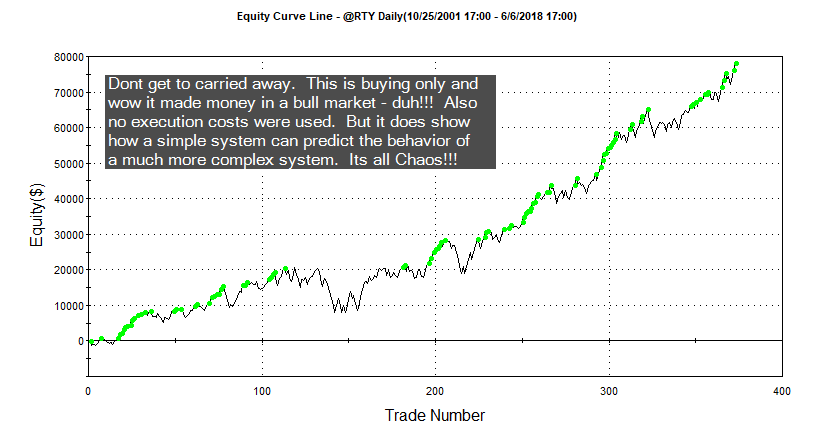Register on the website and I will email you an ELD of the improved Pattern Smasher.  Or just shoot me an email.

# How to Create a Dominant Cycle Class in Python

John Ehlers used the following EasyLanguage code to calculate the Dominant Cycle in a small sample of data.  If you are interested in cycles and noise reduction, definitely check out the books by John Ehlers – “Rocket Science for Traders” or “Cybernetic Analysis for Stocks and Futures.”  I am doing some research in this area and wanted to share how I programmed the indicator/function in Python.  I refer you to his books or online resources for an explanation of the code.  I can tell you it involves an elegantly simplified approach using the Hilbert Transform.

``````Inputs:	Price((H+L)/2);

Vars:	Imult(.635),
Qmult (.338),
InPhase(0),
count(0),
Re(0),
Im(0),
DeltaPhase(0),
InstPeriod(0),
Period(0);

If CurrentBar > 8 then begin
Value1 = Price - Price;
Inphase = 1.25*(Value1  - Imult*Value1) + Imult*InPhase;

//    print(price," ",price," ",value1," ",inPhase," ",Quadrature," ",self.im[-1]," ",self.re[-1])
//	print(d," ",h," ",l," ",c," ",Value1," ",Imult*Value1," ", Imult*InPhase," ",Inphase);
print(d," ",o," ",h," ",l," ",c," ",value1," ",inPhase," ",Quadrature," ",Re," ",Im);
If Re <> 0 then DeltaPhase = ArcTangent(Im/Re);

{Sum DeltaPhases to reach 360 degrees.  The sum is the instantaneous period.}
InstPeriod = 0;
Value4 = 0;
For count = 0 to 50 begin
Value4 = Value4 + DeltaPhase[count];
If Value4 > 360 and InstPeriod = 0 then begin
InstPeriod = count;
end;
end;

{Resolve Instantaneous Period errors and smooth}
If InstPeriod = 0 then InstPeriod = InstPeriod;
Period = .25*InstPeriod + .75*Period;

Plot1(Period, "DC");``````
EasyLanguage Code For Calculating Dominant Cycle

In my Python based back tester an indicator of this type is best programmed by using a class.  A class is really a simple construct, especially in Python, once you familiarize yourself with the syntax.   This indicator requires you to refer to historical values to calculate the next value in the equation:  Value1, inPhase, re, etc.,.  In EasyLanguage these values are readily accessible as every variable is defined as a BarArray – the complete history of a variable is accessible by using indexing.  In my PSB I used lists to store values for those variables most often used such as Open, High, Low, Close.  When you need to store the values of let’s say the last five bars its best to just create a list on the fly or build them into a class structure.  A Class stores data and data structures and includes the methods (functions) that the data will be pumped into.  The follow code describes the class in two sections:  1) data declaration and instantiation and 2) the function to calculate the Dominant Cycle.  First off I create the variables that will hold the constant values: imult and qmult.  By using the word self I make these variables class members and can access them using “.” notation.  I will show you later what this means.  I also make the rest of the variables class members, but this time I make them lists and instantiate the first five values to zero.  I use list comprehension to create the lists and zero out the first five elements – all in one line of code.  This is really just a neat short cut, but can be used for much more powerful applications.  Once you create a dominantCycleClass object the object is constructed and all of the data is connected to this particular object.  You can create many dominantCycleClass objects and each one would maintain its own data.  Remember a class is just a template that is used to create an object.

``````class dominantCycleClass(object):
def __init__(self):
self.imult = 0.635
self.qmult = 0.338
self.value1 = [0 for i in range(5)]
self.inPhase = [0 for i in range(5)]
self.quadrature = [0 for i in range(5)]
self.re = [0 for i in range(5)]
self.im = [0 for i in range(5)]
self.deltaPhase = [0 for i in range(5)]
self.instPeriod = [0 for i in range(5)]
self.period = [0 for i in range(5)]``````
Data Portion of Class

The second part of the class template contains the method or function for calculating the Dominant Cycle.  Notice how I index into the lists to extract prior values.  You will also see the word self. preceding the variable names used in the calculations Initially I felt like this redundancy hurt the readability of the code and in this case it might.  But by using self. I know I am dealing with a class member.  This is an example of the ” . ” notation I referred to earlier.  Basically this ties the variable to the class.

``````def calcDomCycle(self,dates,hPrices,lPrices,cPrices,curBar,offset):
tempVal1 = (hPrices[curBar - offset] + lPrices[curBar-offset])/2
tempVal2 = (hPrices[curBar - offset - 7] + lPrices[curBar-offset - 7])/2
self.value1.append(tempVal1 - tempVal2)
self.inPhase.append(1.25*(self.value1[-5] - self.imult*self.value1[-3]) + self.imult*self.inPhase[-3])
if self.re[-1] != 0.0: self.deltaPhase.append(degrees(atan(self.im[-1]/self.re[-1])))
if len(self.deltaPhase) > 51:
self.instPeriod.append(0)
value4 = 0
for count in range(1,51):
value4 += self.deltaPhase[-count]
if value4 > 360 and self.instPeriod[-1] == 0:
self.instPeriod.append(count)
if self.instPeriod[-1] == 0: self.instPeriod.append(self.instPeriod[-1])
self.period.append(.25*self.instPeriod[-1]+.75*self.period[-1])
return(self.period[-1])``````
Dominant Cycle Method

Okay we now have the class template to calculate the Dominant Cycle but how do we us it?

``````#---------------------------------------------------------------------------------
#Instantiate Indicator Classes if you need them
#---------------------------------------------------------------------------------
#    rsiStudy = rsiClass()
#    stochStudy = stochClass()
domCycle = dominantCycleClass()
#---------------------------------------------------------------------------------
#Call the dominantCycleClass method using " . " notation.
tempVal1 = domCycle.calcDomCycle(myDate,myHigh,myLow,myClose,i,0)
#Notice how I can access class members by using " . " notation as well!
tempVal2 = domCycle.imult``````
Dominant Cycle Object Creation

Here I assign domCycle the object created by calling the dominantCycleClass constructor.  TempVal1 is assigned the Dominant Cycle when the function or method is called using the objects name (domCycle) and the now familiar ” . ” notation.  See how you can also access the imult variable using the same notation.

Here is the code in its entirety.  I put this in the indicator module of the PSB.

``````class dominantCycleClass(object):
def __init__(self):
self.imult = 0.635
self.qmult = 0.338
self.value1 = [0 for i in range(5)]
self.inPhase = [0 for i in range(5)]
self.quadrature = [0 for i in range(5)]
self.re = [0 for i in range(5)]
self.im = [0 for i in range(5)]
self.deltaPhase = [0 for i in range(5)]
self.instPeriod = [0 for i in range(5)]
self.period = [0 for i in range(5)]

def calcDomCycle(self,dates,hPrices,lPrices,cPrices,curBar,offset):
tempVal1 = (hPrices[curBar - offset] + lPrices[curBar-offset])/2
tempVal2 = (hPrices[curBar - offset - 7] + lPrices[curBar-offset - 7])/2
self.value1.append(tempVal1 - tempVal2)
self.inPhase.append(1.25*(self.value1[-5] - self.imult*self.value1[-3]) + self.imult*self.inPhase[-3])
if self.re[-1] != 0.0: self.deltaPhase.append(degrees(atan(self.im[-1]/self.re[-1])))
if len(self.deltaPhase) > 51:
self.instPeriod.append(0)
value4 = 0
for count in range(1,51):
value4 += self.deltaPhase[-count]
if value4 > 360 and self.instPeriod[-1] == 0:
self.instPeriod.append(count)
if self.instPeriod[-1] == 0: self.instPeriod.append(self.instPeriod[-1])
self.period.append(.25*self.instPeriod[-1]+.75*self.period[-1])
return(self.period[-1])``````
Dominant Cycle Class - Python

# Mean Reversion Regime Change?

Many traders of late have done quite well buying the dips (Mean Reversion) in the stock market.  I mean if you look at the big picture and just not the last two months.  The hyper aggressive buyers might have bitten off more than they could chew.  The laid back fully funded traders were able to shrug off the volatility – even when the DOW was down nearly quadruple digits some kept going in and buying in a very respectful manner.  Of course they had their protective stops as well as profit objectives in place as soon as their buys went in.  Take a look at this equity curve:The huge draw down in just a matter of a few days shook out a ton of traders – even though the drawdown had probably been seen a few times in the history of the algorithm.   When you buy after the DOW was down 1175 you have to be crazy right?  That’s exactly what a lot of systems did and if you didn’t have a relatively respectable stop then you might have gotten wiped out.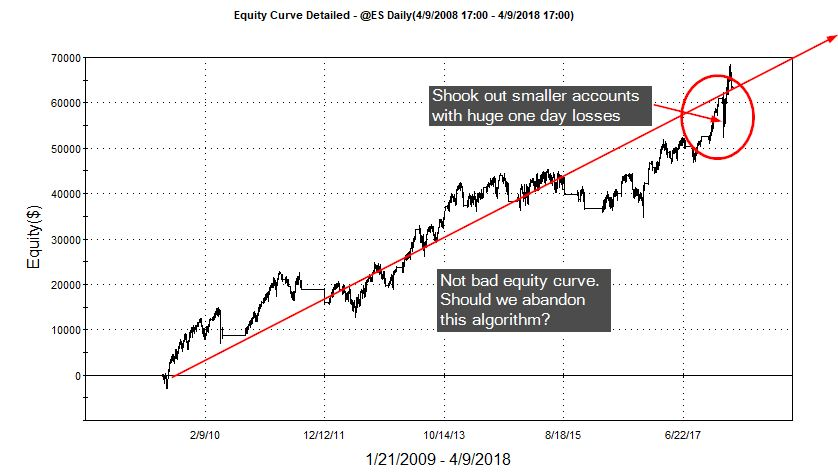This system risks \$1000 to make \$4000 and only buys when you have two lower lows.  Oh and the close has to be above the 200 day moving average.  The intra-trade draw down was around \$6000 during the February Vixpocalypse.  How can that be?  If I am only risking \$1000 why did I lose almost \$6000 on one given trade.

Many of the more popular mean reversion systems fell into this same situation because they utilized a stop on a close basis only.  In other words the market could collapse during the day, but you had to wait to get out on the close.  This can be dangerous even if historical performance has shown respectable performance metrics.  Waiting for the close has been able to turn large losing trades into either much smaller losing trades or even winners.

Here is the same system with an intraday stop of \$2000.  I had to increase the stop to get a similar looking equity curve.  I also expanded the profit objective.  Trading these systems requires a balancing act – do you want the security of a daily fixed stop or the chance at a better looking equity curve.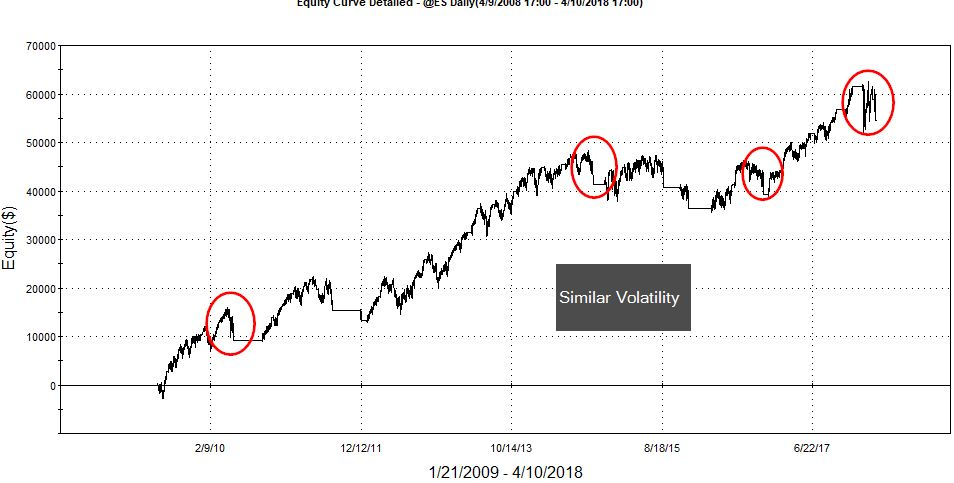A volatility cut off could definitely help with draw down but at the same time its going to bite into the bottom line.  I don’t see a regime change yet.  A fixed stop might provide enough safety to continue trading with these types of algorithms.

# Pyramiding and then Scaling Out at Different Price Levels – EasyLanguage

## TOTAL, TOTAL, TOTAL – an important keyword

I just learned something new!  I guess I never programmed a strategy that pyramided at different price levels and scaled out at different price levels.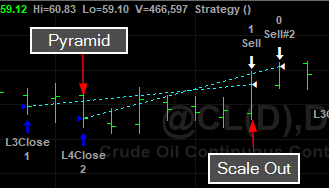Initially I thought no problem.  But I couldn’t get it to work – I tried everything and then I came across the keyword Total and then I remembered.  If you don’t specify Total in you exit directives then the entire position is liquidated.  Unless you are putting all your positions on at one time – like I did in my last post.   So remember if you are scaling out of a pyramid position use Total in your logic.

``````vars: maxPosSize(2);

If currentContracts < maxPosSize - 1 and c > average(c,50) and c = lowest(c,3) then buy("L3Close") 1 contract this bar on close;
If currentContracts < maxPosSize and c > average(c,50) and c = lowest(c,4) then buy("L4Close") 1 contract this bar on close;

If currentContracts = 2 and c = highest(c,5) then sell 1 contract total this bar on close;
If currentContracts = 1 and c = highest(c,10) then sell 1 contract total this bar on close;``````
Scaling Out Of Pyramid

Why you have to use the Total I don’t know.  You specify the number of contracts in the directive and that is sufficient if you aren’t pyramiding.  The pyramiding throws a “monkey wrench” in to the works.

# First Put Multiple Contracts On:

``If c > average(c,200) and c = lowest(c,3) then buy("5Large") 5 contracts this bar on close;``
Using keyword contracts to put on multiple positions

Here you specify the number of contracts prior to the keyword contracts.

Easylanguage requires you to create a separate order for each exit.  Let’s say you want to get out of the 5 positions at different times and possibly prices.  Here’s how you do it:

``````If currentContracts = 5 and c > c then sell 1 contracts this bar on close;
If currentContracts = 4 and c > c then sell 1 contracts this bar on close;
If currentContracts = 3 and c > c then sell 1 contracts this bar on close;
If currentContracts = 2 and c > c then sell 1 contracts this bar on close;
If currentContracts = 1 and c > c then sell 1 contracts this bar on close;``````
One order for each independent exit

The reserved word currentContracts hold the current position size.  Intuitively this should work but it doesn’t.

{If currentContracts > 0 then sell 1 contract this bar on close;}

You also can’t put order directives in loops.  You can scale out using percentages if you like.

``````Value1 = 5;

If currentContracts = 5 and c > c then sell 0.2 * Value1 contracts this bar on close;
If currentContracts = 4 and c > c then sell 1 contracts this bar on close;
If currentContracts = 3 and c > c then sell 1 contracts this bar on close;
If currentContracts = 2 and c > c then sell 1 contracts this bar on close;
If currentContracts = 1 and c > c then sell 1 contracts this bar on close;``````
Using a percentage of original order size

That’s all there is to scaling out.  Just remember to have an independent exit order for each position you are liquidating.  You could have just two orders:  scale out of 3 and then just 2.

# Setting Stop Loss and Profit Target Utilizing EntryPrice with EasyLanguage

## One Problem with the “Next Bar” Paradigm – market position nor entryPrice are adjusted by the end of the bar

Whenever I develop a strategy I like to program all of my entries and exits without utilizing TradeStations built-in execution functions.  I just got use to doing this when I started programming in Fortran many years ago.  However, there a few scenarios where this isn’t possible.  If you enter a trade and use the following logic to get you out with a loss or a profit when referencing your entryPrice, you will be surprised with your results.  This is because you are telling the computer to use entryPrice before you know what it is.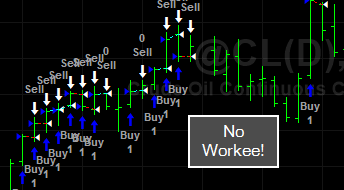This logic is absolutely correct in its intention.  However, TradeStation doesn’t realize you are in a position at the end of the bar and can’t properly reference entryPrice.  Okay so we force TradeStation to only issue orders once it has a valid entryPrice.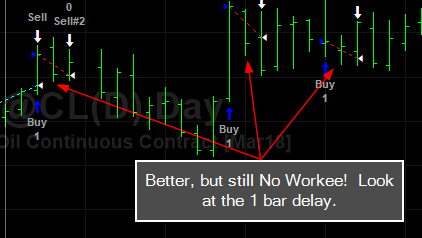TradeStation only realizes the correct marketPosition the following day and then issues an order for the next bar.  So we get the one bar delay.  It would be helpful if TradeStation would set the marketPosition at the close of the bar on the bar of entry.   However, you can overcome this with TradeStation’s built-in execution functions.  For some reason these functions know exactly when you get in – you can also get the same results by inserting the respective strategies on the chart.

## An Easy Fix ThoughBut this little bug can creep into other areas of your programming.  Keep an eye on this.

# Using TradeStation’s Development Environment Projects

I have started using the TDE’s projects more and more.  Before, when I was working on multiple related files I would lose track of them in the editor tabs, or discover, when working over time on different projects, I would have twenty or thirty files open – this of course would slow the editor down.  Using projects can clean up your workspace and speed up productivity.  Projects have been around forever and most professional IDEs  incorporate them to aid in the organization and productivity of the programmer.

Here is simple Project with three components:  indicator, function, and strategy.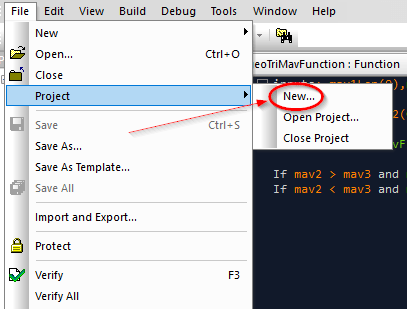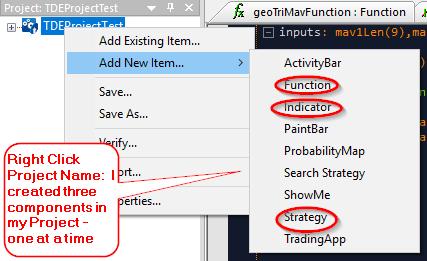Notice how you can focus just on the components of the project.I also like to split the editor window and populate the bottom pane with code I might need to refer.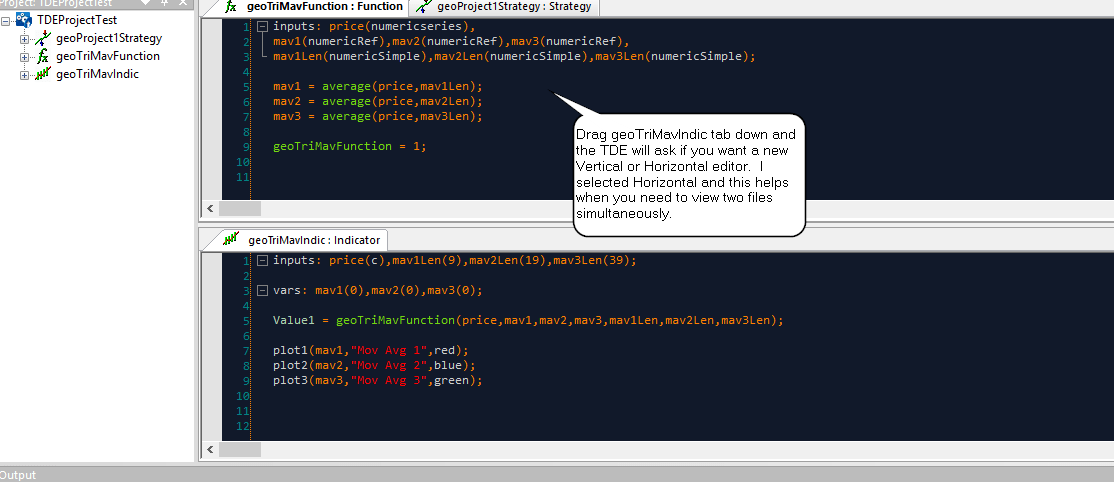Check out the multiple output function – geoTriMavIndic.

# Re-Entry After Taking A Profit

Here is some code I have been working on.  I will go into detail on the code a little later.  But this is how you monitor re-entering at a better price after taking a profit.  The problem with taking profits on longer term trend following systems is that the logic that got you into the position is probably still true and you will notice your algorithm will re-enter in the same direction.  So you need to inform your algorithm not to re-enter until a certain condition is met.  In this example, I only re-enter at a better price if the condition that got me into the trade is still valid.

``````Inputs: swingHiStrength(2),swingLowStrength(2),numDaysToLookBack(30),stopAmt\$(500),profitAmt\$(1000),getBackInAfterProfAmt\$(250);
vars: mp(0),longProfTaken(false),shortProfTaken(false);

mp = marketPosition;

if not(longProfTaken) and mp <> 1 then Buy("BreakOut-B") next bar at highest(h,20) on a stop;
if not(shortProfTaken) and mp <>-1 then SellShort("BreakOut-S") next bar at lowest(l,20)  on a stop;

//If mp = 0 and mp = 1 then print(date," ",exitPrice(1)," ",entryPrice(1));

If longProfTaken then
Begin
If c < exitPrice(1) - getBackInAfterProfAmt\$/bigPointValue then
begin
longProfTaken = false;
If mp <> -1 then buy("longRe-Entry") next bar at open;
end;
end;

If shortProfTaken then
Begin
If c > exitPrice(1) + getBackInAfterProfAmt\$/bigPointValue then
begin
shortProfTaken = false;
If mp <>-1 then sellShort("shortRe-Entry") next bar at open;
end;
end;

If mp = 1 and c > entryPrice + profitAmt\$/bigPointValue then
begin
sell this bar on close;
longProfTaken = true;
end;

If mp =-1 and c < entryPrice - profitAmt\$/bigPointValue then
begin